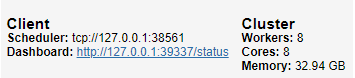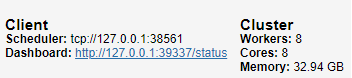# How To: Panel with worker thread

Hi all,

It took me a while to get around the document lock issues, but I’ve found a way to supply a panel app with a worker-thread that gets live-data.
Others will probably also deal with this issue, so I decided to show my approach:

Notebook 1 - Pub

``````import pandas as pd
import numpy as np
from streamz import Stream
from dask.distributed import Client, Pub, Sub
import warnings
warnings.filterwarnings('ignore')

client = Client(n_workers=8)
pub = Pub('test',client=client)
client
``````

This will create a dask pub/sub stream, which you can use to distribute your data:Copy, the scheduler port. You will need it for the 2nd script.

Now let’s define some processing pipeline to transform data, using the streamz library (you can also directly use pub.put(), if you won’t do any processing):

``````def _put(x):
pub.put(x)

# Some pipeline to be defined
source = Stream()
source.sink(_put)
``````

Finally, let’s put some stuff in our stream:

``````for i in range(100):
# Sending random dataframes with x,y,z
x = np.random.rand(1)
y = np.random.rand(1)
z = np.random.rand(1)
source.emit(pd.DataFrame({"x":x,"y":y,"z":z}).set_index("x"))
``````

Notebook 2 - Sub (panel app)

``````import holoviews as hv
import pandas as pd
import numpy as np
import panel as pn
from panel.io import unlocked
from holoviews.streams import Pipe, Buffer
from dask.distributed import Client, Pub, Sub

hv.extension('bokeh')

# Use the same scheduler
client = Client("tcp://127.0.0.1:38561")
sub = Sub('test',client=client)
client
``````Now, let’s define our buffer & plot:

``````# Example df for buffer
df = pd.DataFrame({"x":np.array([]),
"y":np.array([]),
"z":np.array([])}).set_index("x")

buffer = Buffer(data=df)

# Some example scatter plot
dmap = hv.DynamicMap(hv.Scatter, streams=[buffer]).opts(bgcolor='black', color='z', show_legend=False)
``````

We still need to get the data from the pub/sub stream, so let’s put them in a local buffer, called ‘temp’:

``````# Store pub/sub result in local buffer
temp = []

# Panel object
p1 = pn.Column(dmap)

# Function to put things into plot buffer
def to_buffer():
with unlocked():
while len(temp):
msg = temp.pop()
buffer.send(msg)

# Function to pop elements from pub/sub stream
def _pop():
# This for-loop will continously try to pop elements
for msg in sub:
temp.append(msg)
# Print length of pub/sub stream
print(f"{len(sub.buffer):<20}",end="\r")

# Launch separate thread for pub/sub
worker.start()
``````

Finally, let’s add a periodic callback with the default of 500ms frequency, and make the app servable.

``````# Periodic call to update the plot
p1.servable()
``````

Serve with panel serve:

`panel serve --port 5006 --ssl-certfile cert.pem --ssl-keyfile private.key --allow-websocket-origin app.materialindicators.com --num-procs 8 Dask_sub.ipynb`

Results:

Let’s load the app on multiple windows to see what users would see:

Trigger the data generation in the first notebook with this cell:

``````for i in range(100):
# Sending random dataframes with x,y,z
x = np.random.rand(1)
y = np.random.rand(1)
z = np.random.rand(1)
source.emit(pd.DataFrame({"x":x,"y":y,"z":z}).set_index("x"))
``````

The GIF below shows the results in action

That’s all. I hope others will find it useful too!

3 Likes

For me this is already very usefull. Thanks so much @Material-Scientist.I think this is a very important example. If by any means you/ we/ someone could take it even further and get it into the official documentation or the Gallery i think it would help Panel a lot.

And if it could be extended to showcase how to extract, transform and plot live data from some external, live, streaming source like a REST api or Kafka that would be awesome.

1 Like

For the record there is an alternative approach here Can i load data asynchronously in Panel?.

If someone understands the pros and cons of these methods they would be nice to know.

Very nice! I didnt know about that pub/sub system.
Both implementations are quite similar where they both use a dask Client to do the asynchronous work.
I think the differences are that the pub/sub system is more suitable with higher refresh rate applications, while I’m only using a dask client where I submit a large calculation and I’m not really concerned about the final result updating very quickly.

But I do have an application in mind which will require faster updates so that might be a good opportunity to try the pub/sub system.

2 Likes

Thanks @Material-Scientist , @Jhsmit and @Marc . I suppose both Dask Client approaches should run faster than a simpler add_next_tick_callback approach? In bokeh discourse they suggest to use the add_next_tick_callback. I tried to perform similar task of this post.

``````import panel as pn, numpy as np
import holoviews as hv, pandas as pd
import asyncio
import time

from functools import partial
from holoviews.streams import Pipe, Buffer

pn.extension()
pn.config.sizing_mode = 'stretch_width'
hv.extension('bokeh')

# Example df for buffer
df = pd.DataFrame({"x":np.array([]),
"y":np.array([]),
"z":np.array([])}).set_index("x")

buffer = Buffer(data=df)

# Some example scatter plot
dmap = hv.DynamicMap(hv.Scatter,
streams=[buffer]).opts(bgcolor='black',
color='z',
show_legend=False,
width = 1200, height = 800,responsive=False
)

@asyncio.coroutine
def update(x,y,z):
buffer.send(pd.DataFrame({"x":x,"y":y,"z":z}).set_index("x"))

for i in range(100):
x = np.random.rand(1)
y = np.random.rand(1)
z = np.random.rand(1)
# update the document from callback
if doc:
else:
print('if not working', i)

def button_click(event):

btn = pn.widgets.Button(name='Run')
btn.on_click(button_click)

p1 = pn.Column(btn,
pn.Row(dmap,  width = 1200, height = 800, sizing_mode="fixed")
)
p1.show('streaming hv')
``````

Should i learn Dask or do the same thing?? What is the advantage of moving towards using dask despite its greater complexity?

2 Likes

The dask will only run faster if you have really big computational tasks, because there the work can be done in ~10 (depending on your CPU) parallel processes, rather than threads. But this does give a lot of overhead, if you have small tasks then you’re probably better off with your current implementation which has a lot less overhead.

For me another reason to use dask is that you can very easily use the same code but run your webapp and your dask cluster on separate machines.
I plan to host my panel application on microsoft azure, but if you host there and choose hardware sufficient for the heavy computation stuff you will pay a lot of money for hardware that most of the time will be doing nothing. So then by separating both you can rent minimal hardware to keep the GUI responsive and then elsewhere on azure host the Dask part where you can pay per CPU/GPU cycle.

But this is all still very much in the stage of this is the plan, I havent yet started putting dask/panel on azure.

3 Likes

For now I am just playing on my laptop but at some point we will implement it on our local server with IIS. I will try to test Dask at that time.

1 Like

Sure, here’s a crude example of live BTC/USDT price data via API:

Just change the emitting code in notebook 1 to this:

``````import requests, json, time

while True:
# Crude example for live data
r = requests.get("https://www.binance.com/api/v3/avgPrice?symbol=BTCUSDT").text
# response only contains avg price of last 5min, so let's add the current timestamp
df = pd.DataFrame({"date":pd.datetime.utcnow(),
"price":r["price"]},index=).set_index("date")
df.price = df.price.astype(np.float64)

# Emit to stream
source.emit(df)

# Wait 50ms due to API limit
time.sleep(0.05)
``````

This will just get the avg price for the last 5min every 50ms

Then, in notebook 2, change the example df to contain the price & date label, with the corresponding dtypes, and use the hv.Curve plot instead:

``````# Example df for buffer
df = pd.DataFrame({"date":np.array([],dtype="<M8[ns]"),
"price":np.array([],dtype=np.float64),
}).set_index("date")

buffer = Buffer(data=df)

# Some example curve plot
dmap = hv.DynamicMap(hv.Curve, streams=[buffer])
``````

Result:

This is the easiest example I could think of for live data via API.
Please let me know how I could help if you want to put this in the gallery/docs.

~Fred

1 Like

Thanks for that example!